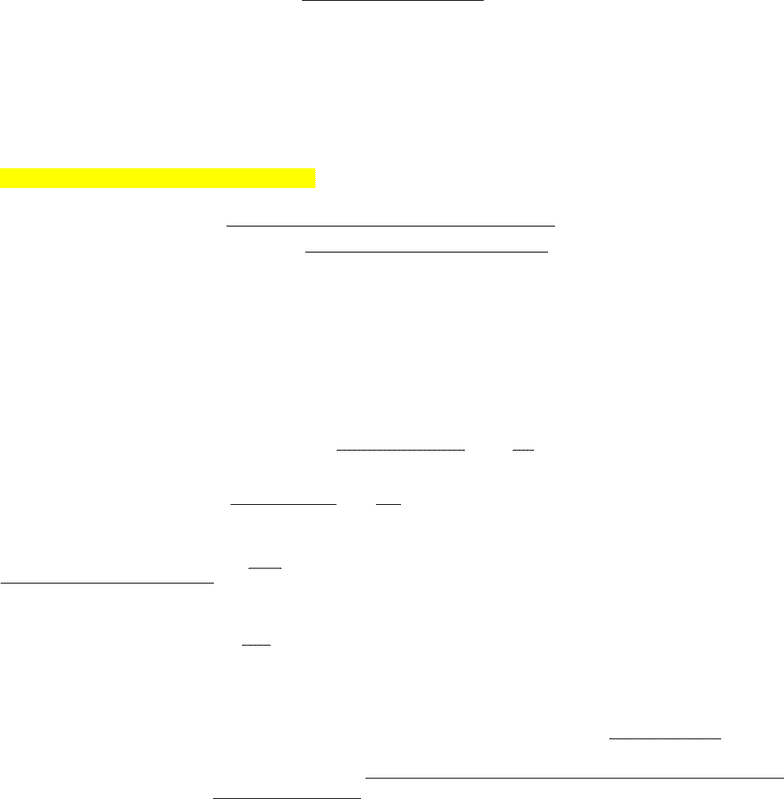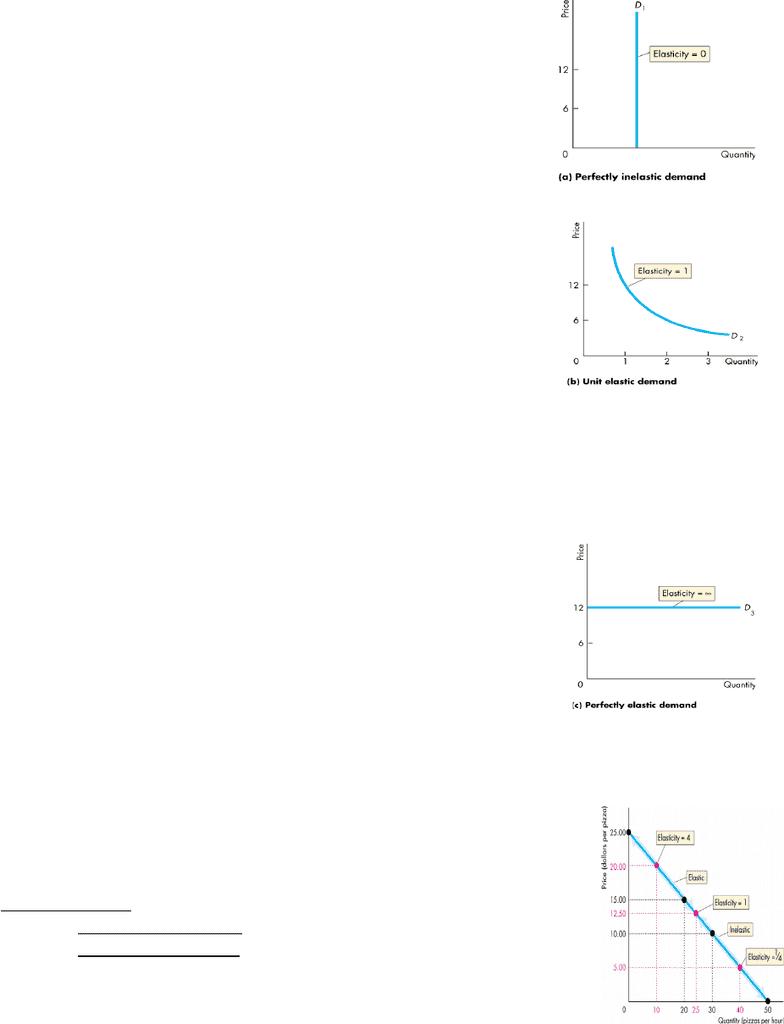# ECON101 Chapter Notes - Chapter 4: Demand Curve, Negative Number, Inferior Good

14 views6 pages
School
Department
Course
ProfessorSept 30, Oct 5 Lecture 6-7 1
Chapter 4: Elasticity
Elasticity:
Measure of degree of responsiveness in certain factors: income, price, supply
Difference lies in demand curve (slope)
oNote: Slope is not elasticity. You can't use slope to make comparisons
Demand curve shows Price and Quantity. The price could be in different currencies. How do
you compare responsiveness in price between CAD and Euros?
Calculating Price Elasticity of Demand
Price Elasticity of Demand: units-free measure of the responsiveness of the quantity demanded of a
good to a change in its price. If price changes, how will quantity change?
Price elasticity of demand =
Ex. \$20.50 a pizza and 9 pizza an hour are demanded. When the price falls to \$19.50 a pizza and
quantity demanded increases to 11 pizzas an hour, what is the Price Elasticity of Demand?
When price falls by \$1/pizza, the quantity demanded increase by 2 pizzas/hour.
Average quantity: 10 and Average price: \$20
Price elasticity of demand =
Q
∆ P
=
20
5
= -4 Interpretation: negative is indication of inverse relationship between
price and quantity demanded, BUT we take it as an absolute value
=4 Interpretation: a 1% decrease in price will lead to a 4% change in
quantity demanded, good is elastic, greater change in quantity demanded
when the price changes
Average Price and Quantity: the midpoint between original price and new price. By using
average price and average quantity, we get the same value for elasticity regardless of whether
the price rises or falls
Units-Free Measure: elasticity is a ratio of two percentage change, so a change in the units
of measurement of price or quantity leaves the elasticity value the same. Allows us to make
comparisons across countries using different kinds of goods
% c h angequantity demanded
% c h angeprice
% change in quantity demanded: %
Q=
quantity c h ange
averagequantity
=
2
10
=
% Change in Price: %
P=
price c h ange
average price
=
= -5%
Unlock document

This preview shows pages 1-2 of the document.
Unlock all 6 pages and 3 million more documents.

Already have an account? Log inSept 30, Oct 5 Lecture 6-7 2
Minus Sign and Elasticity: when price of good rises, quantity demanded decreases. (price
elasticity of demand is a negative number). However, we take absolute value, but interpret it
as inverse relationship
Special case: Prices of ostentation: if prices increase for a certain good, certain people buy
more of it because they want to show off their status
Inelastic and Elastic Demand
Perfectly inelastic demand: if the quantity demanded remains
constant when the price changes
price elasticity of demand is 0
No response to a change in price
a 20% increase in price results in a 0 decrease in quantity
demanded
No matter how high the price goes, I buy the same
amount
Ex. insulin (important for diabetics)
Unit elastic demand: if the percentage change in the quantity
demanded equals the percentage change in the price
price elasticity = 1
1% change in price lead to 1% change in quantity
demanded
Quantity demanded and price change by the same
amount
Inelastic demand: %change in the quantity demanded is smaller than the %change in the
price
Price elasticity between 0-1
10 % change in price leads to a 2% change in quantity demanded
Ex. food and shelter
Perfectly elastic demand: quantity demanded changes by an
infinitely large percentage in response to a tiny price change
Price elasticity=
Consumers are extremely sensitive to a change in price
Ex. Coke from 2 different machines, if the price of Coke
goes up in one machine, no one will buy it from that machine
and buy it from the other machine
Elastic demand: percentage change in the quantity demanded exceeds the percentage
change in price
Price elasticity >1
Ex. automobiles and furniture
Elasticity along a Linear Demand Curve
Elasticity changes as you move along a linear demand curve
At the mid-point of the demand curve, demand is unit elastic.
At prices above the mid-point of the demand curve, demand is elastic.
At prices below the mid-point of the demand curve, demand is inelastic
Total Revenue and Elasticity
Total revenue= price of good * quantity sold
Unlock document

This preview shows pages 1-2 of the document.
Unlock all 6 pages and 3 million more documents.

Already have an account? Log in

# Get access

Grade+
\$10 USD/m
Billed \$120 USD annually
Homework Help
Class Notes
Textbook Notes
40 Verified Answers
Study Guides
1 Booster Class
Class+
\$8 USD/m
Billed \$96 USD annually
Homework Help
Class Notes
Textbook Notes
30 Verified Answers
Study Guides
1 Booster Class